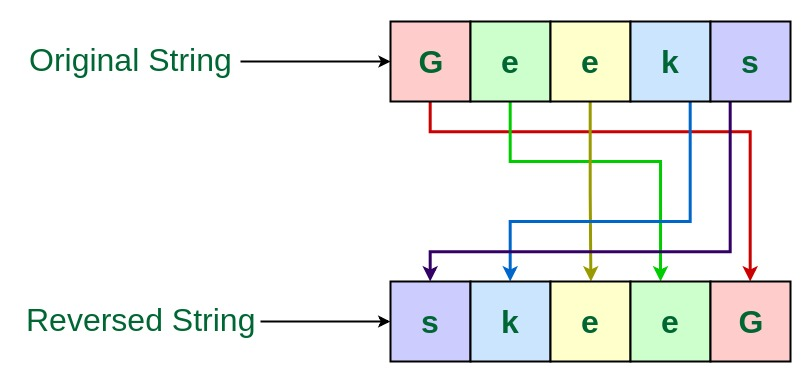Related Articles

# TCS Coding Practice Question | Reverse a String

• Difficulty Level : Medium
• Last Updated : 04 Apr, 2019

Given a string, the task is to reverse this String using Command Line Arguments.

Examples:

```Input: Geeks
Output: skeeG

Input: GeeksForGeeks
Output: skeeGroFskeeG
```Approach 1: Using another String to store the reverse

• Since the string is entered as Command line Argument, there is no need for a dedicated input line
• Extract the input string from the command line argument
• Create a String to store the resultant reversed string, say reversedString
• Traverse through this String character by character using loop, in reverse order
• Now append each character into the resultant reversedString
• This is the required reversed string

Program:

## C

 `// C program to reverse a string``// using command line arguments`` ` `#include ``#include ``#include `` ` `// Function to reverse the String``char``* reverseString(``char` `input[])``{`` ` `    ``// Get the length of the string``    ``int` `length = ``strlen``(input);``    ``int` `i;`` ` `    ``// String to store the reverse``    ``char``* reversedString``        ``= (``char``*)``malloc``(length * ``sizeof``(``char``));`` ` `    ``// Loop through the string``    ``// character by character in reverse order``    ``// and store it into the resultant string``    ``for` `(i = length - 1; i >= 0; i--)``        ``reversedString[length - 1 - i] = input[i];`` ` `    ``// Return the reversed String``    ``return` `reversedString;``}`` ` `// Driver code``int` `main(``int` `argc, ``char``* argv[])``{``    ``// Check if the length of args array is 1``    ``if` `(argc == 1)``        ``printf``(``"No command line arguments found.\n"``);``    ``else` `{`` ` `        ``// Get the command line argument``        ``// and reverse it``        ``printf``(``"%s\n"``, reverseString(argv));``    ``}``    ``return` `0;``}`

## Java

 `// Java program to reverse a string``// using command line arguments`` ` `class` `GFG {`` ` `    ``// Function to reverse the String``    ``public` `static` `String reverseString(String input)``    ``{`` ` `        ``// String to store the reverse``        ``String reversedString = ``""``;`` ` `        ``// Loop through the string``        ``// character by character in reverse order``        ``// and store it into the resultant string``        ``for` `(``int` `i = input.length() - ``1``; i >= ``0``; i--)``            ``reversedString += input.charAt(i);`` ` `        ``// Return the reversed String``        ``return` `reversedString;``    ``}`` ` `    ``// Driver code``    ``public` `static` `void` `main(String[] args)``    ``{`` ` `        ``// Check if length of args array is``        ``// greater than 0``        ``if` `(args.length > ``0``) {`` ` `            ``// Get the command line argument``            ``// and reverse it``            ``System.out.println(reverseString(args[``0``]));``        ``}``        ``else``            ``System.out.println(``"No command line "``                               ``+ ``"arguments found."``);``    ``}``}`

Output:

Approach 2: Without using another String to store the reverse

• Since the string is entered as Command line Argument, there is no need for a dedicated input line
• Extract the input string from the command line argument
• Traverse through this String character by character using loop till half the length of the sting
• Swap the characters from one end with the characters from the other end
• This is the required reversed string

Program:

## C

 `// C program to reverse a string``// using command line arguments`` ` `#include ``#include ``#include `` ` `// Function to reverse a string``char``* reverseString(``char` `str[])``{`` ` `    ``int` `n = ``strlen``(str);``    ``int` `i;``    ``char` `temp;`` ` `    ``// Swap character starting from two``    ``// corners``    ``for` `(i = 0; i < n / 2; i++) {``        ``temp = str[i];``        ``str[i] = str[n - i - 1];``        ``str[n - i - 1] = temp;``    ``}`` ` `    ``return` `str;``}`` ` `// Driver code``int` `main(``int` `argc, ``char``* argv[])``{``    ``// Check if the length of args array is 1``    ``if` `(argc == 1)``        ``printf``(``"No command line arguments found.\n"``);``    ``else` `{`` ` `        ``// Get the command line argument``        ``// and reverse it``        ``reverseString(argv);`` ` `        ``// Print the reversed string``        ``printf``(``"%s\n"``, argv);``    ``}``    ``return` `0;``}`

## Java

 `// Java program to reverse a string``// using command line arguments`` ` `class` `GFG {`` ` `    ``// Function to reverse the String``    ``public` `static` `String reverseString(String str)``    ``{`` ` `        ``int` `n = str.length();``        ``char` `temp;`` ` `        ``// Swap character starting from two``        ``// corners``        ``for` `(``int` `i = ``0``; i < n / ``2``; i++) {``            ``str = str.substring(``0``, i)``                  ``+ str.charAt(n - i - ``1``)``                  ``+ str.substring(i + ``1``, n - i - ``1``)``                  ``+ str.charAt(i)``                  ``+ str.substring(n - i);``        ``}`` ` `        ``return` `str;``    ``}`` ` `    ``// Driver code``    ``public` `static` `void` `main(String[] args)``    ``{`` ` `        ``// Check if length of args array is``        ``// greater than 0``        ``if` `(args.length > ``0``) {`` ` `            ``// Get the command line argument``            ``// and reverse it``            ``System.out.println(reverseString(args[``0``]));``        ``}``        ``else``            ``System.out.println(``"No command line "``                               ``+ ``"arguments found."``);``    ``}``}`

Output:

Want to learn from the best curated videos and practice problems, check out the C Foundation Course for Basic to Advanced C.

My Personal Notes arrow_drop_up